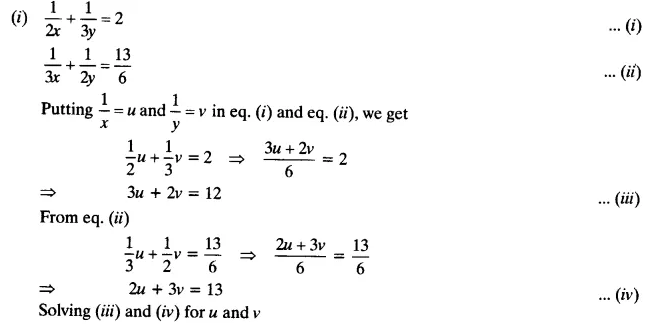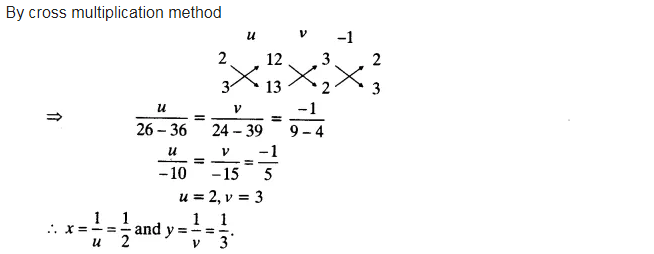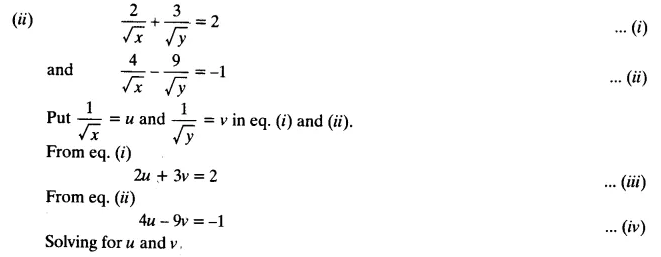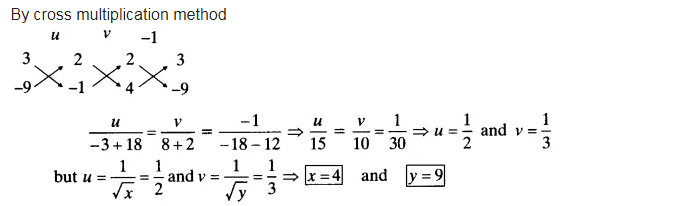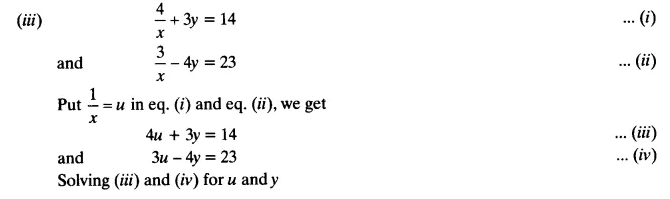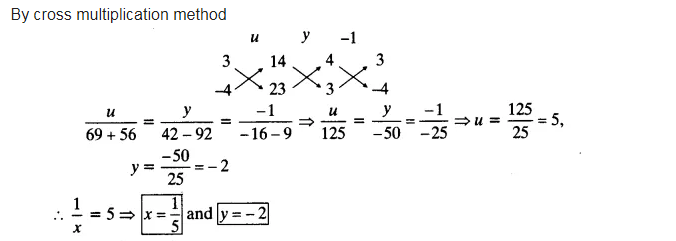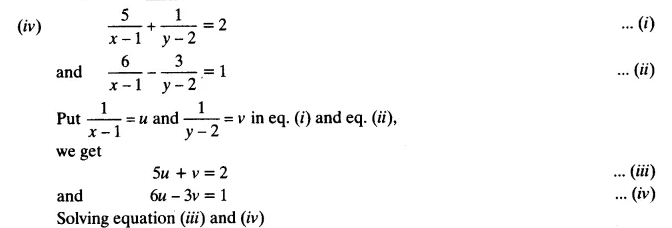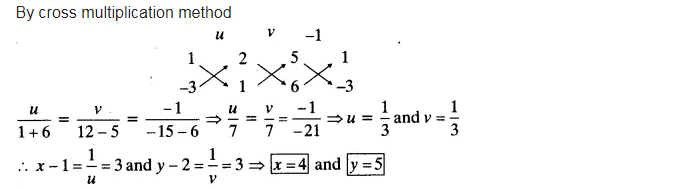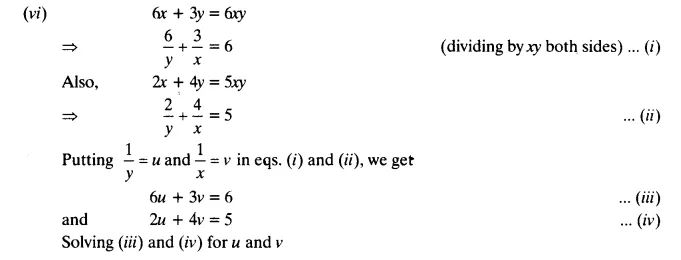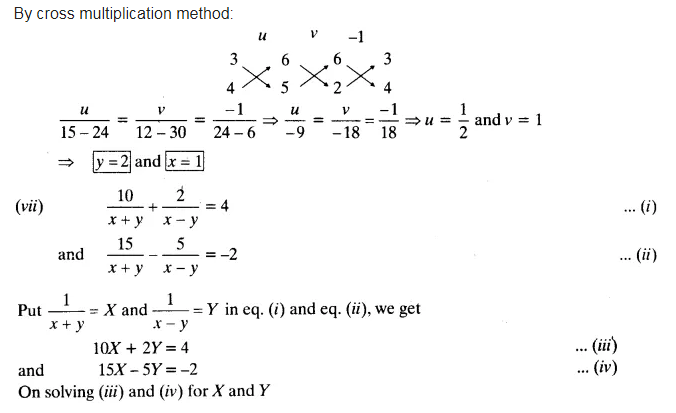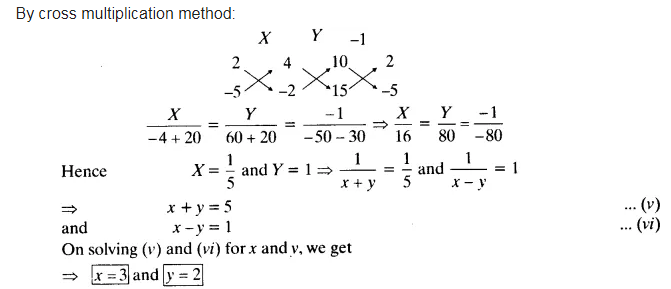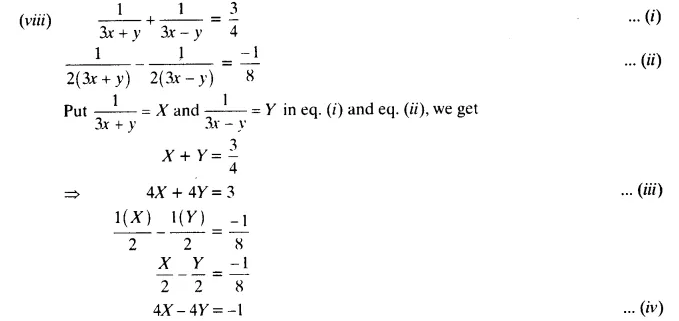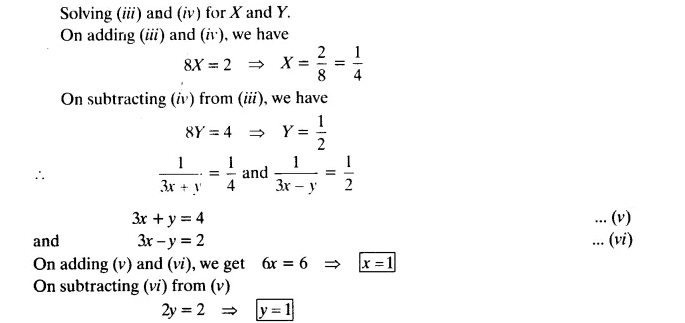# Solve the following pairs of equations by reducing them to a pair of linear equations:

Solve the following pairs of equations by reducing them to a pair of linear equations: